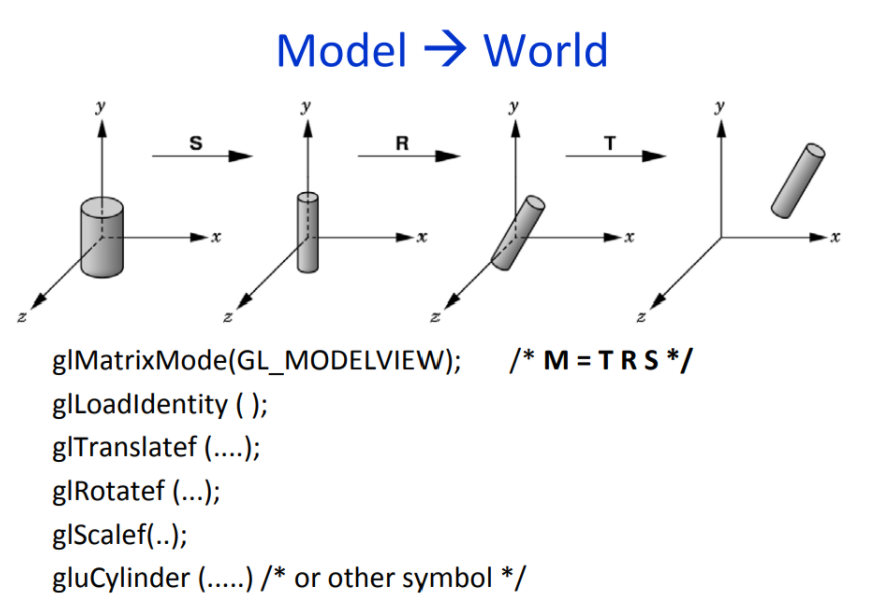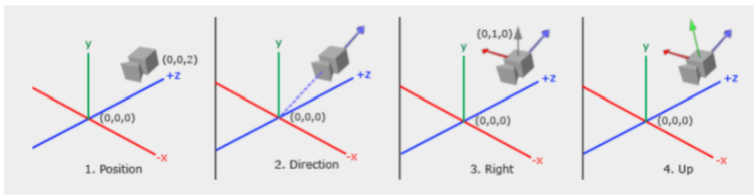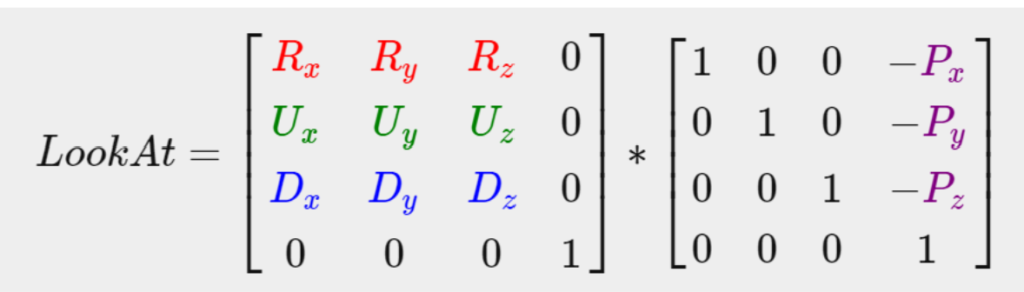# Understanding Various Coordinate Systems in OpenGL

Introduction

My first impression when the term “coordinate systems” came up in my graphic class was that it is easy; everyone knows what a coordinate system is. However, when I started to work on my first project with OpenGL, I started to be overwhelmed by too many coordinate systems. There are at least 5 different important coordinate systems that learners should notice: Object space (or local space, or model space), world space, view space (or eye space, or camera space), clip space, and screen space. If we do not understand this concept well, we might have trouble later as we try to build our first 3D object.

The Right hand coordinate system in OpenGL

The coordinate system used in OpenGL is right hand coordinate. Different from the 3D coordinate that we learned from math, the y axis is up and the positive z axis points towards the viewer. Figure 1 is helpful for me to understand the roles and relationships of those different spaces in the general graphic pipeline .

Local space (or model space) is the coordinate of the object relative to its local origin (0,0,0). For example, initially, a sphere is at the origin, its position can be later moved to a different location using transformation using model matrix. By this process, the object is put into a world space whose coordinates help locate the locations of all objects in the virtual world that is defined by our programming.

Matrix transformation from model space to world space

The process of transforming from model space to world space can be performed by many types of transformation: translation, scaling, and rotation. Let V(x,y,z) is a vertex of the object. Mathematically, to transform object coordinate to world coordinate, we multiply matrices as shown below. Mathematical concepts help learners understand what actually happens when a 3D object transforms. However, in practice, no matrix multiplication is explicitly done by users.  We just simply call built-in functions such as glTranslatef(), glRotatef(), or glScalef() etc.; but we need to know proper parameters to pass in the functions so that objects can end up in the desired location in world space by understanding matrix transformation.

Another important key in this transformation is the order of transformation; this is taken into consideration when compound transformation is done. Compound transformation is a series of transformations; they need to be written in the reversed order before the object is dawn. Basically, compound transportation is matrix transformation; translation and rotation are not commutative. As illustrated in figure 2, rotation after translation create different result from translation after rotation .

Example of transformation done in OpenGL

As shown in the figure 3 , originally, the object coordinate is at (0,0,0). We want the object to be scaled, rotated and then translated into world space. The order of our built-in functions for transformation are arranged in a reversed order: glTranslatef() is called before glRotatef() , and then glScalef() before object is drawn by calling gluCylinder().Figure 3: An example of transformation in OpenGL. Source:http://www.csc.villanova.edu/~mdamian/Past/graphicsfa10/notes/HierarchyHand.pdf

View space is the scene that is viewed by the eye (or camera). In order to set the view space, we need to somehow set the view matrix and perform matrix transformation. In OpenGL, users can simply call the function gluLookAt( ex, ey, ez, lx, ly, lz, ux, uy, uz ). In this function (ex, ey, ez) is the eye position, (lx,ly, lz) is the look-at position, and (ux,uy, uz) is the up vector. This function will automatically do the calculation for users. Some learners might wonder what is the up vector? The up vector is used to calculate the right vector. The picture below (Figure 4) will help users understand more about the eye position, direction, right vector, and up vector.Figure 4: How the camera coordinate is set in OpenGL. Source: Adapted from 

Mathematically, this is also a matrix multiplication as shown in Figure 5. R, U, D are the right vector, the up vector, and the direction vector respectively. P is the eye’s position.Figure 5: Matrix multiplication related to the function gluLookAt(). Source: Adapted from 

Clip space is formed by projecting view space using projection matrix. OpenGL requires x, y, z coordinates of vertices ranging from -1.0 to 1.0 in order to show up on the screen. Otherwise, any vertices that are outside of the clipping space will be clipped. There are two types of projection: orthographic and perspective projection. Figure 6 shows the different visual effect from those projections. The perspective projection imitates how the image is observed by eyes in real life.

In OpenGL, to do the perspective projection, we use the function gluPerspective( fovy, aspect, zn, zf ). Fovy is the vertical angle in degrees; aspect is the width-height ratio; zn is the near clipping plane and zf is the far clipping plane. To do the orthographic projection, we use the function void glOrtho (GLdouble left, GLdouble right, GLdouble bottom, GLdouble top, GLdouble nearVal, GLdouble farVal)

Conclusion:

A solid understanding of how coordinate systems work in the graphic pipeline is important as learners might apply that concept when building their first 3D object. Matrix transformation is the basic mechanism for moving object space into world space. It is helpful to relate built-in functions in OpenGL with their works in different spaces related to the graphic pipeline.

Reference

 Learning OpenGL -Your #1 Resource for OpenGL. Coordinate System.[Online]. Available: https://learnopengl.com/Getting-started/Coordinate-Systems. Accessed on Oct 1st, 2021.

 OpenGL Programming Guide. Appendix F Homogeneous Coordinates and Transformation Matrices. [Online]. Available: https://www.glprogramming.com/red/appendixf.html. Accessed on Oct 1st, 2021.

 Zeyang Li. Transformations in OpenGL. Carnegie Mellon University. [Online]. Available: https://www.cs.cmu.edu/afs/cs/academic/class/15462-s09/www/lec/03/lec03a.pdf. Accessed on Oct 2nd, 2021.

 Villanova University. 3D OpenGL Basic Models Hierarchical Modeling. [Online]. Available: http://www.csc.villanova.edu/~mdamian/Past/graphicsfa10/notes/HierarchyHand.pdf. Accessed on Oct 2nd, 2021